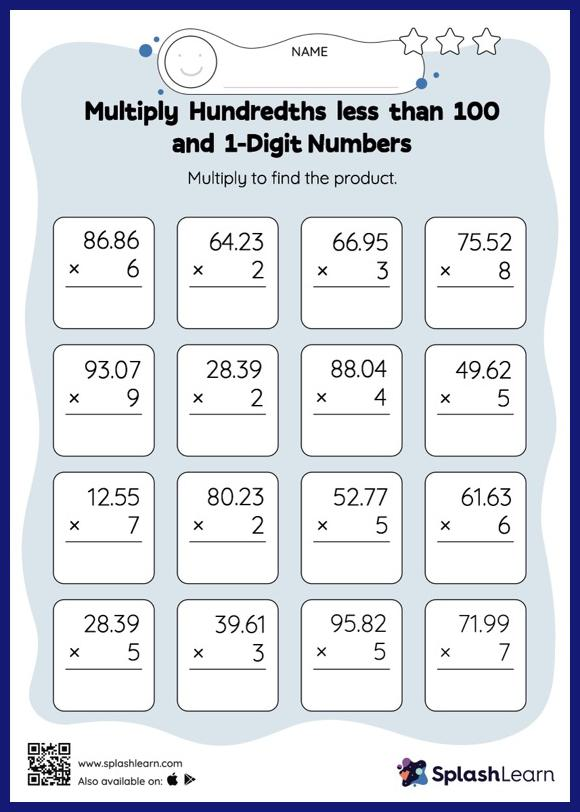# Multiply Hundredths less than 100 and 1-Digit Numbers: Vertical Multiplication Worksheet

Home > Multiply Hundredths less than 100 and 1-Digit Numbers: Vertical MultiplicationStudents may omit the decimal point when multiplying a decimal by a one-digit number and then add it when writing the answer. This multiply hundredths less than 100 and 1-digit numbers worksheet gives learners many opportunities to practice this concept. In this worksheet, numbers are laid one on top of another (vertical format). This encourages students to use the inherent place value structure to solve the problems.